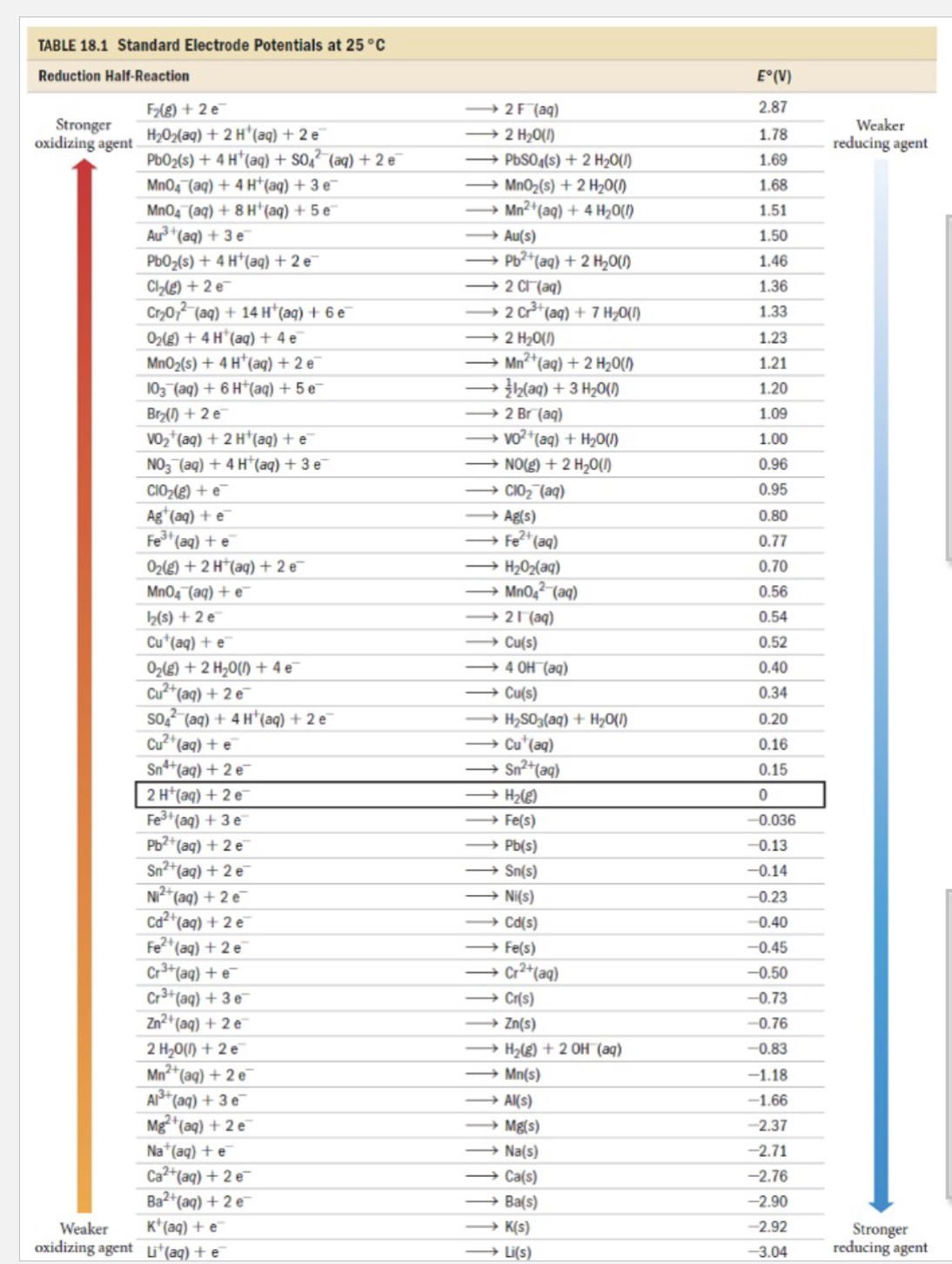Home / Expert Answers / Chemistry / write-balanced-equations-for-the-reactions-that-each-of-the-following-species-might-undergo-in-aerat-pa960

# (Solved): Write balanced equations for the reactions that each of the following species might undergo in aerat ...

Write balanced equations for the reactions that each of the following species might undergo in aerated aqueous acid (pH = 0). If the species is stable, write ‘no reaction’: Justify your answers. (use table to rationzalie concepts)TABLE 18.1 Standard Electrode Potentials at Write balanced equations for the reactions that each of the following species might undergo in aerated aqueous acid . If the species is stable, write 'no reaction': Justify your answers. (a) (b) (c) Note: You need to consider that there are 2 oxidants present in "aerated aqueous acid", and .

We have an Answer from Expert